HomeAudienceDevelopersBe Cautious while using Bit Fields for Programming

# Be Cautious while using Bit Fields for Programming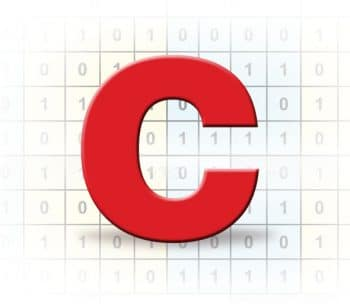In this article, the author tells embedded C programmers why writing code involving bit fields needs to be done carefully, failing which the results may not be as expected. However, bit fields are handy as they involve only low level programming and result in efficient data storage.

Embedded C programmers who have worked with structures should also be familiar with the use of bit fields structures. Use of bit fields is one of the key optimisation methods in embedded C programming, because these allow one to pack together several related entities, where each set of bits and single bits can be addressed. Of course, the usage of bit fields is easy’ and comes handy, especially during low level programming. Though considered as one of the unique features of C programming, bit fields do have some limitations. Let us look at these by exploring the example problems in this article.

Data types and bit fields
Let us look into the signed qualifiers affecting the output of the bit field structure. Please note that the code snippets provided here are tested with the GCC compiler [gcc version 4.7.3] running under a Linux environment.
Let us consider a simple small C code snippet as shown below, with a structure named bit field, with three integer fieldshours, mins and secs, of bit field sizes 5, 6 and 6, respectively:

typedef struct bit_field
{
int hours : 5;
int mins : 6;
int secs : 6;
}time_t;

Now let us declare a variable alarm of type time_t and set values as 22, 12 and 20, respectively:

//Declaration of the variable of type time_t
time_t alarm;
/Assigning the values to the different members of the bit-field structures
alarm.hours = 22;
alarm.mins  = 12;
alarm.secs  = 20;

When we print these values using a simple printf statement, what could be the output? At first, most of us will envision the answers to be 22, 12 and 20, for hours, mins and secs respectively. Whereas when we actually compile and run the run the code, the value printed for the hours would be different – 10, 12 and 20 (as shown in Figure 1).

Where did we go wrong?
1. We all know that the default signed qualifier for the int’ is signed int’.
2.     We reserved 5 bits for storing the hours field assuming we were using the 24-hour format. From among 5 bits, 1 bit was used for storing the sign of the number, which means only 4 bits were then available for storing the actual value. In these 4 bits, we can store the numbers ranging from -16 to +15 according to the formula (-2^k) to ([+2^k] -1)) including 0, where  k’  indicates the number of bits.

3.    We will see how 22 is stored in binary form in 5 bits through pictorial representation (Figure 2).
4.    From the table(as shown in Figure 2), it is very clear that sign bit (b4) is SET, which indicates the value is negative. So, when printed using the printf statement, we will get -10 (the decimal value of 10110), because of which we got an unexpected output.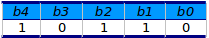Figure 2 : Pictorial representation of binary value of 24 in ‘5’ bits

Now that we have understood the problem, how do we fix it? It is very simple; just qualify int to unsigned int just before the hours in the bit field structure, as shown below. The corrected output is shown in Figure 3.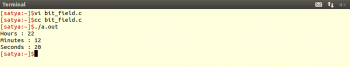Figure 3 : Correct output after correct usage of datatype

#include <stdio.h>
typedef struct bit_field
{
unsigned int hours  : 5;
unsigned int mins   : 6;
unsigned int secs    : 6;
}time_t;
int main()
{
//Declaration of the variable of type time_t
time_t alarm;
//Assigning the values to the different members of the bit-field structures
alarm.hours = 22;
alarm.mins  = 12;
alarm.secs  = 20;
printf(“Hours : %d\nMins : %d\nSecs : %d\n”, alarm.hours, alarm.mins, alarm.secs);
}

Bit wise operators definitely provide advantages, but they need to be used a bit carefully. In the embedded programming environment, they might lead to major issues in case they are not handled properly.

Endianess of the architecture and bit fields
In this problem, we will see how Endianess affects the bit fields. Bit fields in C always start at Bit 0, which is the least significant bit (LSB) on Little Endian. But most compilers on Big Endian systems inconveniently consider the most significant bit (MSB)Bit 0.
Note: Big Endian machines pack bit fields from the most significant byte to the least significant.

Little Endian machines pack bit fields from the least significant byte to the most.
To start with, let us consider the code( Labelled as byte_order.c) given below:

1 #include <stdio.h>
2 typedef union {
3         unsigned int value;
4         struct {
5                 unsigned char one   : 8;
6                 unsigned char two   : 8;
7                 unsigned char three : 8;
8                 unsigned char four  : 8;
9         } bit_field;
10 } data_t;
11
12 int main() {
13
14         data_t var = {0x1A1B1C1D};
15         unsigned char *ptr = (unsigned char *)(&var);
16
17         printf(“The entire hex value is 0x%X\n”, var.value);
18         printf(“The first byte is 0x%X @ %p\n”, *(ptr + 0), ptr + 0);
19         printf(“The second byte is 0x%X @ %p\n”, *(ptr + 1), ptr + 1);
20         printf(“The third byte is 0x%X @ %p\n”, *(ptr + 2), ptr + 2);
21         printf(“The fourth byte is 0x%X @ %p\n”, *(ptr + 3), ptr + 3);
22
23         return 0;
24 }

When I run this code in my system, I get the output shown in Figure 4.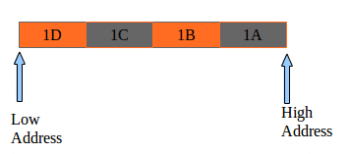Figure 5 : Byte-ordering in Little – Endianess Machine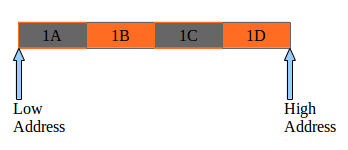Figure 6 : Byte-ordering in Big – Endianess Machine

From Figure 4, it is very clear that the underlying architecture is following the little Endian. When the same code is run under a different architecture, which follows Big Endian, the result will be different. So, portability issues need to be considered while using bit fields.
Lets look at one more example to understand how bits are packed in Big Endian and Little Endian.
To start with, let us consider the sample code(Labelled as bit_order.c) given below:

1 #include <stdio.h>
2 typedef union {
3         unsigned short value;
4         struct {
5                 unsigned short v1   : 1;
6                 unsigned short v2   : 2;
7                 unsigned short v3   : 3;
8                 unsigned short v4   : 4;
9                 unsigned short v5   : 5;
10         } bit;
11 } data_t;
12
13 int main() {
14
15         data_t var ;
16         unsigned char *ptr = (unsigned char*)(&var);
17         var.bit.v1   = 1;
18         var.bit.v2   = 2;
19         var.bit.v3   = 3;
20         var.bit.v4   = 4;
21         var.bit.v5   = 5;
22
23         printf(“The Entire hex value is 0x%X\n”, var.value);
24         printf(“The first byte is 0x%X @ %p\n”, *(ptr + 0), ptr + 0);
25         printf(“The second byte is 0x%X @ %p\n”, *(ptr + 1), ptr + 1);
26
27         return 0;
28 }

When I run this code in my system, I get the output as shown in Figure 7.

From this figure, one can see that the bits are packed from the least significant on a little Endian machine. Figure 8 helps us understand how the bits ordering takes place.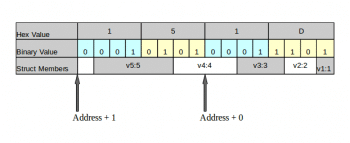Figure 8 : Bit – ordering in small Endianess architecture

If you run the same code in big Endian architecture, you will get the output given in Figure 9.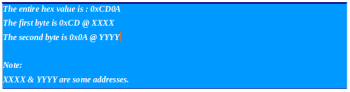Figure 9 : Expected output of the code bit_order.c when run in big-endian architecture.

For more clarity, see Figure 10.
From the last two examples, it is very clear that bit fields pose serious portability issues. When the same programs are compiled on different systems, they may not work properly. This is because some C compilers use the left-to-right order, while other C compilers use the right-to-left order. They also have architecture-specific bit orders and packing issues.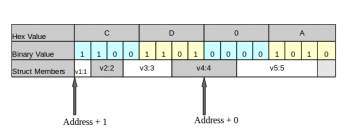Figure 10 : Bit- ordering in Big Endianess Architecture

As a concluding note, let us list the advantages and limitations of bit fields structures.
1.     Efficiency – Storage of data structures by packing.
2.     Readability – Members can be easily addressed by the names assigned to them.
3.     Low level programming  The biggest advantage of bit fields is that one does not have to keep track of how flags and masks actually map to the memory. Once the structure is defined, one is completely abstracted from the memory representation as in the case of bit-wise operations, during which one has to keep track of all the shifts and masks.

Limitations
1.     As we saw earlier, bit fields result in non-portable code. Also, the bit field length has a high dependency on word size.
2.    Reading (using scanf) and using pointers on bit fields is not possible due to non-addressability.
3.    Bit fields are used to pack more variables into a smaller data space, but cause the compiler to generate additional code to manipulate these variables. This results in an increase in both space as well as time complexities.
4. The sizeof() operator cannot be applied to the bit fields, since sizeof() yields the result in bytes and not in bits.Satyanarayana Sampangi
The author is a member of the embedded software team at Emertxe Information Technologies. His area of interest lies in embedded C programming combined with data structures and microcontrollers. He likes to experiment with C programming in his spare time to explore new horizons. He can be reached at satya@emertxe.com.

1.nagarjuna

good…..information…….

2.nagarjuna

but..the figures are not displayed properly……when i click on the figures…..it will directly…went to home page…

3.Parth Mehta

In figure 10, position of “address + 0” and “address + 1” should be interchanged.

4.Sravan Kumar

Good article.